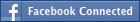• NEW! FREE Beat The GMAT QuizzesHundreds of Questions Highly Detailed Reporting Expert Explanations
• 7 CATs FREE!
If you earn 100 Forum Points

Engage in the Beat The GMAT forums to earn
100 points for $49 worth of Veritas practice GMATs FREEVERITAS PRACTICE GMAT EXAMS Earn 10 Points Per Post Earn 10 Points Per Thanks Earn 10 Points Per Upvote ## Right triangle problem tagged by: ##### This topic has 8 member replies For right triangle RST, RS and RT are legs and RT+RS=12. ST is hypotenuse with length 10. What is the area of the right triangle? A) 24 B) 11 C) 15 D) 2*sqrt(30) E) 12 Newbie | Next Rank: 10 PostsJoined 09 Aug 2009 Posted: 3 messages Is it B Senior | Next Rank: 100 PostsJoined 28 Jan 2010 Posted: 97 messages Upvotes: 9 mattocks wrote: For right triangle RST, RS and RT are legs and RT+RS=12. ST is hypotenuse with length 10. What is the area of the right triangle? A) 24 B) 11 C) 15 D) 2*sqrt(30) E) 12 RS + ST = 12 using pythagoras theorem 100 = x^2 + (x-12)^2 solving we get X as 6+sqrt(14) and 6-sqrt(14) Area = 1/2(6+sqrt(14) and 6-sqrt(14)) = 11 Hence B Master | Next Rank: 500 PostsJoined 05 Jul 2010 Posted: 324 messages Followed by: 3 members Upvotes: 70 Target GMAT Score: 720+ Area of triangle = 1/2 x RS x RT Now, ST^2 = RS^2 + RT^2 RS^2 + RT^2 = (RS+RT)^2 - (2xRTxRS) Thus, ST^2 = (12)^2 - 2xRTxRS => 2RTxRS = 100 - 144 => RT x RS = 22 => 1/2 x RT x RS = 11 This, answer is (B) In other words, (hypotenuse)^2 = (sum of sides)^2 - (4x area of triangle) Junior | Next Rank: 30 PostsJoined 07 Mar 2010 Posted: 11 messages A circle is circumscribed about an equilateral triangle of side 'a'. The diameter of the circle is? A. a*root3 B. a/root3 C. 2a/root3 D. 2*root3(3a) E. 3root3(a) Senior | Next Rank: 100 PostsJoined 22 Jun 2010 Posted: 36 messages Upvotes: 1 GMAT Score: 760 mattocks wrote: A circle is circumscribed about an equilateral triangle of side 'a'. The diameter of the circle is? A. a*root3 B. a/root3 C. 2a/root3 D. 2*root3(3a) E. 3root3(a) Option (C). Circumradius = (2/3 * length of altitude). We can remember this as a rule. Junior | Next Rank: 30 PostsJoined 11 Jul 2010 Posted: 12 messages Upvotes: 2 Target GMAT Score: 730+ GMAT Score: 630 Hey there, my answer is C. Diameter of circumscribed circle of any triangle is abc/(2*area of triangle), where a, b, c are the sides of the triangle. 1. We know that all the sides are equal and length = a 2. To find the area of the triangle, use the 30 60 90 triangle rule, whereby the height will be (root3*a)/2 Therefore, the area of the triangle = 1/2 base* height = 1/2*a*(root3*a)/2) = (a*a*root3)/4 3. Diameter= (a*a*a)/((a*a*root3)/4) = 2a/root3 [/u] Senior | Next Rank: 100 PostsJoined 10 May 2010 Posted: 51 messages Upvotes: 4 Target GMAT Score: 750 kmittal82 wrote: Area of triangle = 1/2 x RS x RT Now, ST^2 = RS^2 + RT^2 RS^2 + RT^2 = (RS+RT)^2 - (2xRTxRS) Thus, ST^2 = (12)^2 - 2xRTxRS => 2RTxRS = 100 - 144 => RT x RS = 22 => 1/2 x RT x RS = 11 This, answer is (B) In other words, (hypotenuse)^2 = (sum of sides)^2 - (4x area of triangle) Now that is an interesting approach and the takeaway is really helpful, thanksLegendary MemberJoined 29 Aug 2009 Posted: 758 messages Followed by: 2 members Upvotes: 67kmittal82 wrote: Area of triangle = 1/2 x RS x RT Now, ST^2 = RS^2 + RT^2 RS^2 + RT^2 = (RS+RT)^2 - (2xRTxRS) Thus, ST^2 = (12)^2 - 2xRTxRS => 2RTxRS = 100 - 144 => RT x RS = 22 => 1/2 x RT x RS = 11 This, answer is (B) In other words, (hypotenuse)^2 = (sum of sides)^2 - (4x area of triangle) Nice solution without any approximation.. _________________ I am on a break !! •5 Day FREE Trial Study Smarter, Not Harder Available with Beat the GMAT members only code •Free Practice Test & Review How would you score if you took the GMAT Available with Beat the GMAT members only code •1 Hour Free BEAT THE GMAT EXCLUSIVE Available with Beat the GMAT members only code •5-Day Free Trial 5-day free, full-access trial TTP Quant Available with Beat the GMAT members only code •Free Veritas GMAT Class Experience Lesson 1 Live Free Available with Beat the GMAT members only code •Award-winning private GMAT tutoring Register now and save up to$200

Available with Beat the GMAT members only code

•Magoosh
Study with Magoosh GMAT prep

Available with Beat the GMAT members only code

•Free Trial & Practice Exam
BEAT THE GMAT EXCLUSIVE

Available with Beat the GMAT members only code

•FREE GMAT Exam
Know how you'd score today for \$0

Available with Beat the GMAT members only code

•Get 300+ Practice Questions

Available with Beat the GMAT members only code

### Top First Responders*

1Ian Stewart 43 first replies
2Brent@GMATPrepNow 35 first replies
3Scott@TargetTestPrep 33 first replies
4Jay@ManhattanReview 28 first replies
5GMATGuruNY 19 first replies
* Only counts replies to topics started in last 30 days
See More Top Beat The GMAT Members

### Most Active Experts

1Scott@TargetTestPrep

Target Test Prep

149 posts
2Max@Math Revolution

Math Revolution

93 posts
3Brent@GMATPrepNow

GMAT Prep Now Teacher

53 posts
4Ian Stewart

GMATiX Teacher

52 posts
5GMATGuruNY

The Princeton Review Teacher

32 posts
See More Top Beat The GMAT Experts